# Electronics and Communication Engineering - Analog Electronics

6.

In a negative feedback amplifier A = 100, β = 0.04 and Vs = 50 mV, then feedback will be

 A. 4 B. 8 C. 10 D. 2

Explanation:

Feed back factor (βA) = 4.

7.

For an npn transistor connected as shown in the figure VBE = 0.7 volts. Given that reverse saturation current of the junction at room temperature 300K is 10-13A, the emitter current.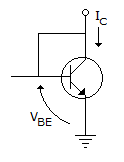A. 30 mA B. 39 mA C. 49 mA D. 20 mA

Explanation:

IE = aIE + ICBO and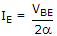approximated.

But a is not given in the question. It is not possible to find.

8.

The dc output voltage of the circuit is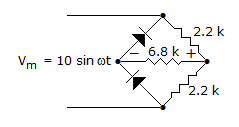A. 4.3 V B. 1.369 V C. 3.87 V D. 1.94 V

Explanation:

This is half wave Rectifier, because in +ve cycle both the diode will be in forward biased.

For +ve cycle,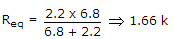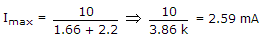Vo max = Imax. Req2.59 x 10-3 x 1.66 x 1034.299 volt.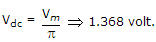9.

A voltage doubler circuit is fed by a voltage Vm sin ωt. The output voltage will be nearly 2 Vm only if

 A. load resistance is small B. load resistance is large C. load resistance is neither small nor large D. either (a) or (c)

Explanation:

If load resistance is large, current would be low and voltage drop would be low.

10.

The purpose of connecting a coupling capacitor in the output circuit of an amplifier is

 A. to increase voltage gain B. to increase collector current C. to block dc collector voltage D. all of the above